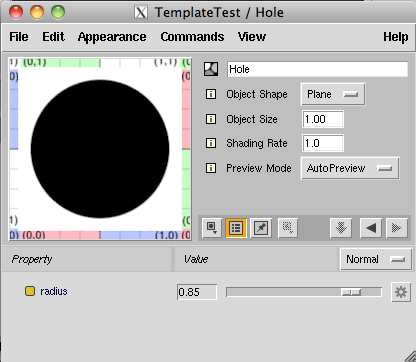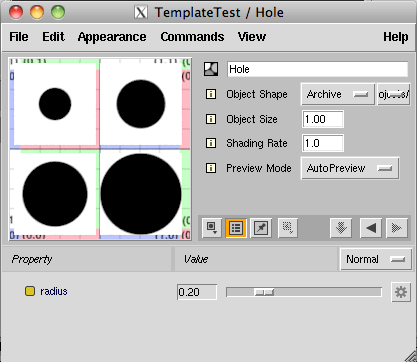### RMSPrimvars & Custom Slim Nodes

#### Introduction

This tutorial provides an example of how a primvar can be read by a custom Slim node (implemented by a custom Slim template). The reader should refer to the tutorial "RIB/RSL: Using Primvars" for basic information about primvars.

To enable the Slim template (listing 2) to be conveniently tested, an archive rib file was assigned to the Slim preview - an overview of using archives with Slim can be found in the tutorial "RMS: Custom Slim Preview". The archive rib file is shown below.

Listing 1 (squares.rib)

 ```AttributeBegin Scale .5 .5 .5 TransformBegin Translate -0.55 0.55 0 Polygon "P" [-0.5 -0.5 0 -0.5 0.5 0 0.5 0.5 0 0.5 -0.5 0] "float radius" [.2] Translate 1.05 0 0 Polygon "P" [-0.5 -0.5 0 -0.5 0.5 0 0.5 0.5 0 0.5 -0.5 0] "float radius" [.3] TransformEnd TransformBegin Translate -0.55 -0.55 0 Polygon "P" [-0.5 -0.5 0 -0.5 0.5 0 0.5 0.5 0 0.5 -0.5 0] "float radius" [.4] Translate 1.05 0 0 Polygon "P" [-0.5 -0.5 0 -0.5 0.5 0 0.5 0.5 0 0.5 -0.5 0] "float radius" [.5] TransformEnd AttributeEnd```

#### An Example Template

Figure 1 shows the template previewing a "Plane". Because the "Plane" does not have a float primvar named "radius" (shown in red above) the slider is able to adjust the size of the black circle.Figure 1 (geometry with without a primvar)

Figure 2 shows the template previewing the archive. Because the polygons each have a "radius" primvar the slider has no effect on the size of their black circles. Instead, the size of the circles is determined by the value of the primvar that is associated with each poly.Figure 2 (surfaces with primvars)

#### The Template

In particular note the use of the RSL function `readprimvar()`. It enables the template to decide if a primvar called "radius" is available for use.

Listing 2 (Hole.slim)

 ```slim 1 extensions cutter { extensions fundza cutr { template float Hole { parameter float radius { detail varying default 0.5 subtype slider range {0 1 0.05} } parameter float result { display hidden access output } RSLFunction { void cutrHole ( float radius; output float result; ) { float rad; if(readprimvar("radius", rad) == 0) rad = radius; point p = transform("object", P); point o = transform("object", point "object" (0,0,0)); float dist = distance(p, o); result = smoothstep(rad - 0.01, rad + 0.01, dist); } } } } }```